Wonderlic : Completing numerical sequences

Example Questions

Example Question #1 : Completing Numerical Sequences

10, 15, 22.5, 33.75

What is the next number in the given sequence?

52.54

48.78

67.5

50.63

50.63

Explanation:

The numbers in the given sequence increase in a uniform pattern, namely that half of the previous number is added to each successive number. Half of 33.75 is 16.88, added to 33.75 this gives us the correct answer: 50.63 (which was also the only answer that ended with the appropriate final decimal number.

Example Question #1 : Completing Numerical Sequences

Give the next number in the sequence: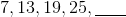Explanation:

To form the sequence, begin with the term 7. Each subsequent term is equal to 6 added to the previous term, as follows: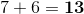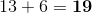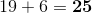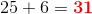,

the missing term.